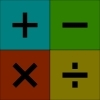#### You may also like### Worms

Place this "worm" on the 100 square and find the total of the four squares it covers. Keeping its head in the same place, what other totals can you make?### Which Scripts?

There are six numbers written in five different scripts. Can you sort out which is which?### Highest and Lowest

Put operations signs between the numbers 3 4 5 6 to make the highest possible number and lowest possible number.

# Sorting the Numbers

##### Age 7 to 11Challenge Level

What do you know about the number $1$, for example? So where would you put it in the diagram?
You could look at each number in turn in this way.

In the second Carroll diagram, what do all the numbers in the top left box have in common?
How are they different to the numbers in the bottom left box, for example?